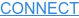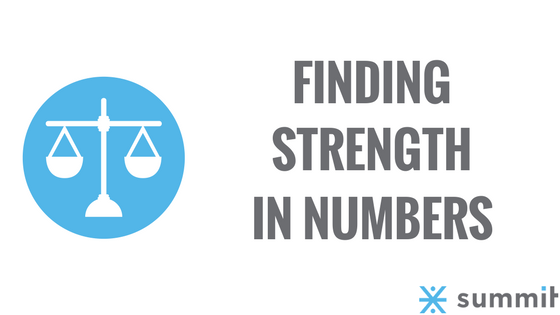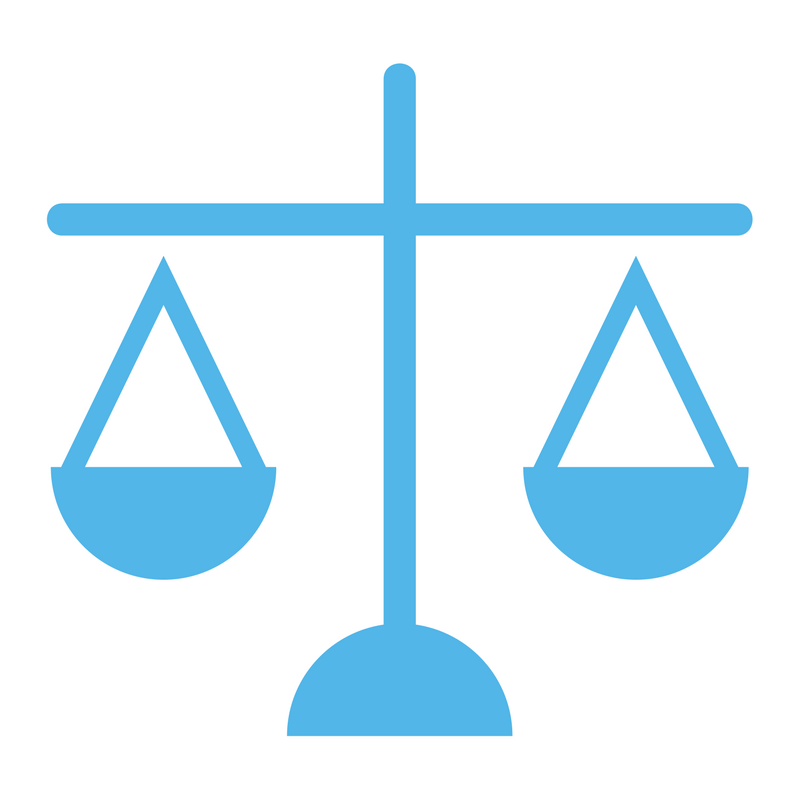In the first post of this blog series on how to use statistics in litigation cases, Dr. Albert Lee and Dr. Shane Thompson discuss finding strength in numbers.At a particularly critical juncture at the Nomura trial, Judge Denise Cote famously quipped “you know, some things don’t lie. There’s math [for example].” This is emblematic of an era when evidence is quantitative and fairness means statistical parity. To be effective advocates in the court room, litigators are increasingly required to fashion their arguments in statistical terms. They must either affirmatively present relevant and compelling statistical evidence, or identify logical and methodological flaws of evidence in rebuttal. Correspondingly, statisticians and economists are becoming vital collaborators for legal counsel.

The purpose of this blog series is to summarize several statistical techniques that have proven consequential in court proceedings. In this first post of the series, we introduce regression analysis.

Statistics are powerful because they summarize a vast amount of data into a small number of metrics, which assist in reaching conclusions. Well-chosen statistics, backed by methodological best practices, allow factfinders to draw conclusions efficiently from data, freeing them from tedious subject-by-subject comparisons. Statistics allow factfinders to see the proverbial forests in the trees.

Reliable statistics possess two important qualities. First, a reliable statistic is unbiased. That is, on average, the statistic is a truthful representation of what it claims to measure. Also, an unbiased statistic is not more likely to overstate than to understate what it claims to measure.

Second, a reliable statistic is precise. Note that a statistic is selected from many possible values, and is often in the “center” of those values. A statistic is precise when possible values are near or cluster around the statistic itself. Put differently, it is imprecise if possible values are spread out.

In well-controlled randomized trials (think lab rats), simple statistics (e.g., averages and medians) may be sufficient to draw conclusions. Litigious settings are not well-controlled trials (think people with different backgrounds and motivations), and simple statistics are often insufficient. These real-life complexities necessitate additional analyses before statistics can produce reliable conclusions. Regression analysis is a well-established technique to handle these real-life complexities.

Regression analysis is a powerful tool that predicts and explains an outcome of interest. The outcome of interest, or “dependent variable,” depends on several factors, or “independent variables.” Regression analysis effectively isolates the contribution of each independent variable holding all other independent variables equal. In other words, regression analysis disentangles the contribution of any one independent variable on the dependent variable from the contributions of all other independent variables. Correspondingly, regression analysis is an indispensable tool in demonstrating parity, forecasting outcomes, and calculating but-for scenarios.

Liability determination. Consider a hypothetical case of pay equity. Suppose that when we compare male and female salaries without accounting for any other factors, we find that males have higher average salaries. Digging deeper, we notice that average levels of education, experience, and training are higher for males. With a regression model, we can control for all factors simultaneously and see if the original pay inequity still remains after accounting for different qualifications. In other words, regression models answer the following question – if males and females at the company had the exact same qualifications, would pay inequity still exist?

Forecasting. Now consider a scenario where we must predict salaries. We don’t observe salaries for certain employees, so we need to predict them using observed salaries from other employees. In this situation, a regression model would estimate the relationship between salary and employee characteristics (i.e., education, experience, training) using salary data for employees that we observe. The characteristics of the employees (for which salary is unobserved) are input into the model to predict their salaries. In this way, regression models allow us to predict outcomes when they do not exist.

But-for scenarios. Suppose that employers in a certain industry colluded to fix wages at an artificially low level for a period of time (call it a collusion period). Regression models could use an indicator variable to identify the collusion period, and quantify wage changes due to collusion (holding fixed other factors). By removing the collusive effect during the collusion period, we could estimate what wages would have been but-for collusion.

Regression analysis is a very powerful tool, but it is only a tool. Expert economists and statisticians must be engaged to implement it effectively. In dynamic matters with complicated data, economists and statisticians can design and substantiate models that produce numbers that “do not lie.” Often, those numbers are the difference between winning and losing.

 Fed. Hous. Fin. Agency v. Nomura Holding Am., Inc., No. 11 Civ. 6201 (S.D.N.Y.)

----------------------------------------------------------------------------------------------------

In the next post in this series, Dr. Lee and Dr. Thompson discuss the use of regression when discussing pay inequity.